### Measuring distances in astronomy

Measuring distance is one of the key facets of astronomical work.

It allows us to gauge the relative brightness of events, allows us to understand the scale of the solar system, our galaxy and the wider universe and these estimates feed in essential parts of our theories about how the universe began and how it may end.

Measurements within the solar system and to nearby stars have a high degree of accuracy and reliability but the more distant the object, the less reliable are the methods and the measurements they make. There remains a substantial margin of error in estimates made using Hubbleʼs law - particularly since there is no absolute agreement on the value of the constant (Hubbleʼs constant) relating speed of recession to distance.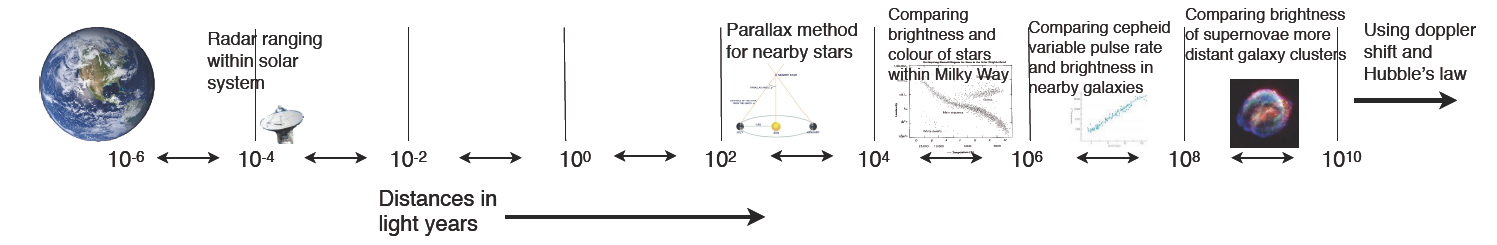Astronomers use a number of different units of measurement, depending on the application. The metre is the standard "Earth bound" unit of length but these and kilometres are too small to avoid use huge numbers which are hard to understand. Indeed all distances in astronomy are hard to understand.

The metre is the base unit of measurement to which all others are finally compared. The definition has undergone a number of changes. It once was 1/10,000 of the distance between the equator and north pole

on a line passing through Paris, but that was hard to standardise. For many years it was the length between two notches of a metal bar held at zero degrees Celsius and is now defined in terms of the speed of light (see www.wikipedia.org for detail).

### The Parsec

The Parsec is a unit very commonly used by astronomers and derived from the AU. It is based upon the parallax method of measuring distances. The change of the angle of observation of a star is measured against a background of other stars at some time in the year and then again six months later. In that time the Earth will be 2AU from itsʼ starting point. If, from the geometry, the angle marked in blue is 1 second (that is 1/3600 of a degree) then the distance to the star is one parsec.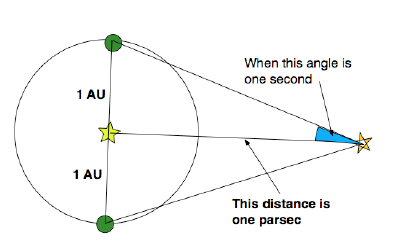The diagram is very misleading. The angle marked in blue should be incredibly small. One second of a degree is 1/60 of a minute of a degree which is 1/60 of a degree. There are 3,600 seconds in one degree and 1,296,000 in a whole circle.

### The Astronomical Unit

The Astronomical Unit (AU) is defined as the average distance from the Earth to the Sun. Since this varies significantly (the Earthʼs orbit is slightly elliptical and irregular) there is International agreement that

1 AU = 149,597,870,691 ± 30 metres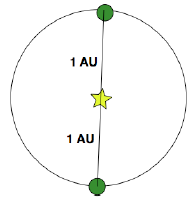An Astronomical Unit 1Au

### A light year

The “Light Year” is the most commonly quoted distance measurement, although maybe not amongst astronomers. It is simply the distance that light would travel in one year. This definition requires a precise definition of one year, in this case a Julian year which is 365.25 days exactly. The light year is then 31,557,600 seconds multiplied by the speed of light at 299,792,458 m/s which gives 9,460,730,472,580.8 kilometres.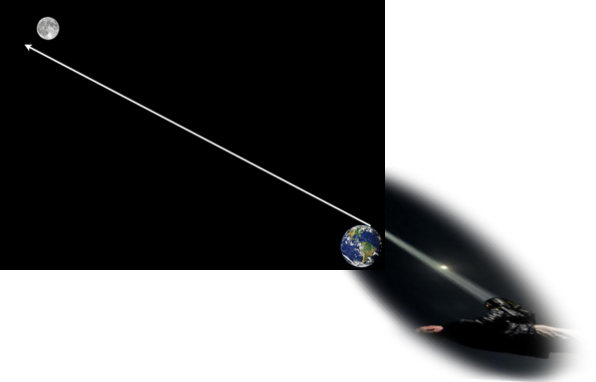It takes just over 1 second for light to travel between the Earth and the Moon

In a terrestrial setting the speed of light is phenomenally fast. Even to the Moon light travels in a little over a second and out of the planetary solar system in a few hours.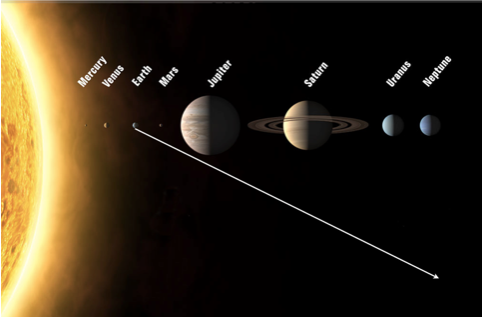It takes light about 8 minutes to travel from the Sun to the Earth and about 4 hours to get as far as Neptune

For large distances, for example across our galaxy, even light years give us a big number. Between galaxies the distances are such the we use units of millions or even billions of light years or parsecs.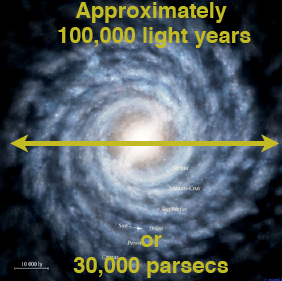The width of our galaxy, The Milky Way

Other pages of notes and video on astronomy which may be useful are:

Measuring distance by parallax/triangulation notes and video     Life cycle of stars    Geostationary and polar satellites notes and video     Big Bang theory and evidence     Development of the Universe after the Big Bang      Real and apparent magnitude     Hubble's Law and measuring distance notes and video     The age of the universe notes and video     Using Hertzsprung Russell diagrams notes and video     Cepheid variable stars      Type 1A supernova